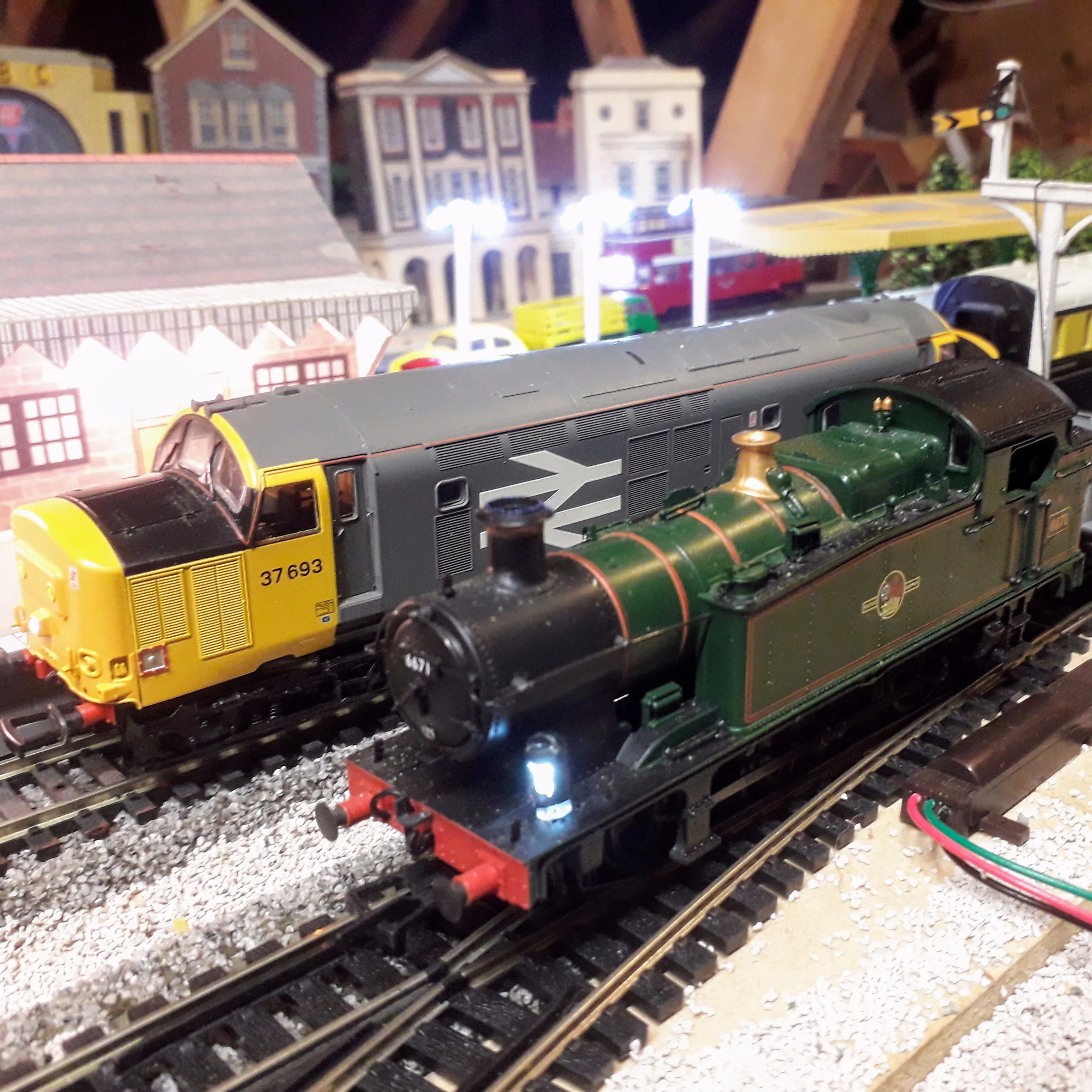# Model Rail Digital DCC Command Station Arduino and Free App

No need to purchase any propriety systems, this is a stand alone unit operated by a free App called 'LocoMotive'

BeginnerFull instructions provided2 hours11,704## Things used in this project

### Hardware components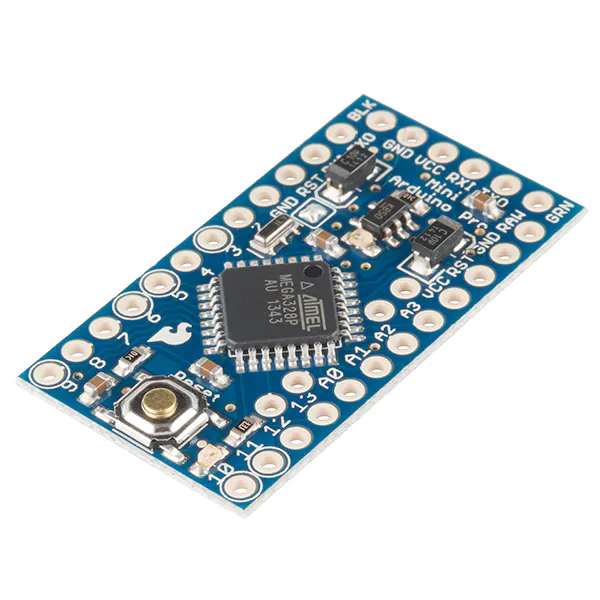SparkFun Arduino Pro Mini 328 - 5V/16MHz
×1
 LMD18200t
×1
 PCB
×1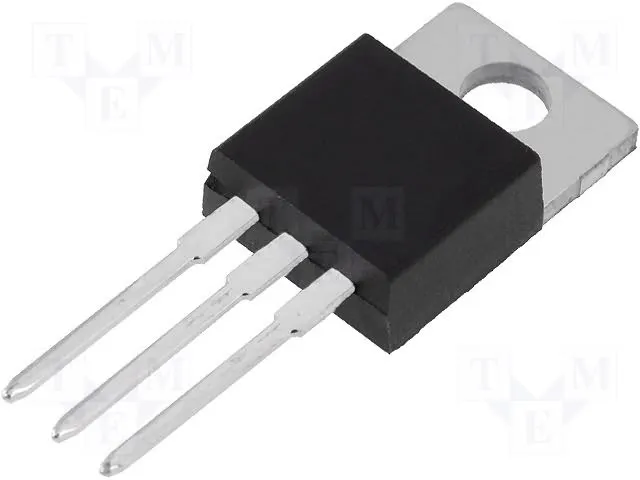Linear Regulator (7805)
×1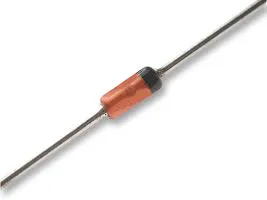3.6V 0.5W Zener Diode
×1
 HC-06 Bluetooth Module
×1
 0.1 ohm 2 watt resistor
×1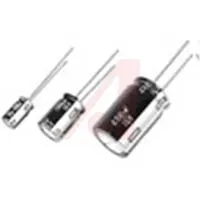Capacitor 220 µF 16 volt
×1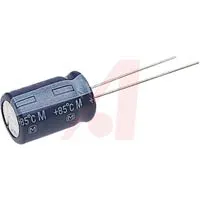Capacitor 10 µF 16 volt
×1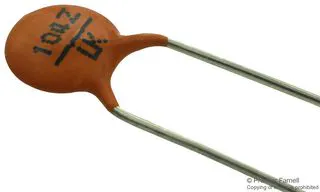Ceramic Disc Capacitor, 0.1 µF
×3Resistor 10k ohm
×2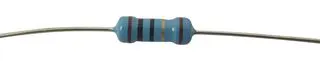Through Hole Resistor, 4.7 kohm
×1
 screw terminal 2 position 3.5 mm centres, 1.1 mm pin dia
×1
 screw terminal 6 position 2.54 mm centres
×1
 4 pin 2.54 mm socket
×1
 12 pin 2.54 mm socket
×2

### Software apps and online services

 LocoMotive This is the free version of the App

## Custom parts and enclosures

### Enclosure

Complete circuit within enclosure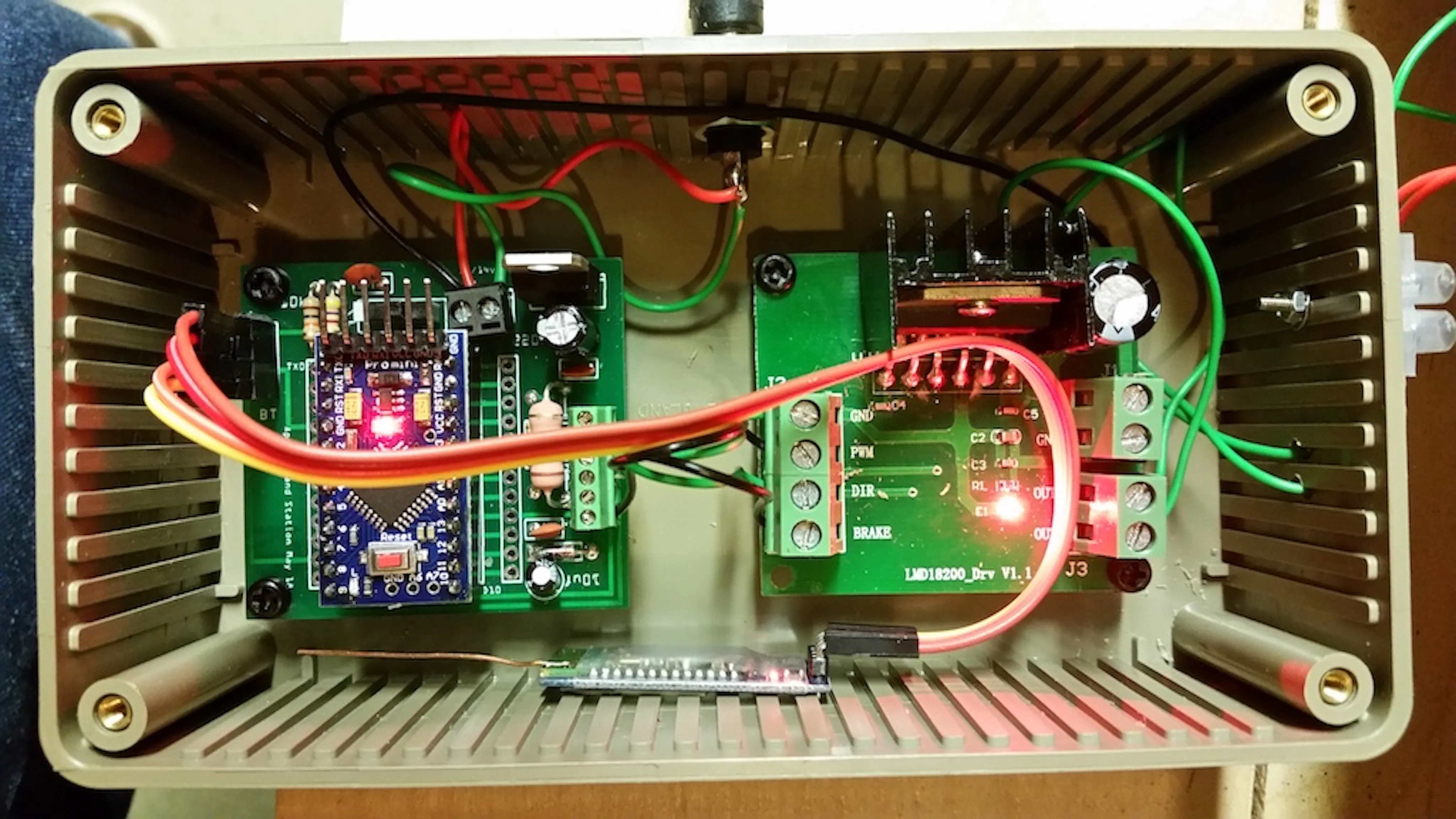## Schematics

### Circuit diagram

Bluetooth receiver / H-Bridge amplifier circuit

App instructions

## Code

### locomotive_dcc_sep19.ino

Arduino
Arduino Code
```// 1 Sep 2019
// sketch for use with Android App 'Locomotive DCC'using LMD18200 h-bridge

#include <SoftwareSerial.h>

SoftwareSerial bluetooth(8,9);  // RX TX
int C;
int sensorValue;
long t = 0;
long lastmillis = 0;
long interval = 1000;
String inString;
int a;
int preamable_type = 0;
float CV_VAL;
float cv_val;
float cv_val0;
float cv_val1;
float cv_val2;
float cv_val3;
float cv_val4;
float cv_val5;
float cv_val6;
float cv_val7;
int test_num;
int cv_write_val;
int Cs;
boolean cv_logic;
boolean ok;
int num;

// use digital pins 6 and 5 for DCC out

//Timer frequency is 2MHz for ( /8 prescale from 16MHz )
#define TIMER_SHORT 0x8D  // 58usec pulse length
#define TIMER_LONG  0x1B  // 116usec pulse length

unsigned char last_timer=TIMER_SHORT;  // store last timer value

unsigned char flag=0;  // used for short or long pulse
unsigned char every_second_isr = 0;  // pulse up or down

// definitions for state machine
#define PREAMBLE 0
#define SEPERATOR 1
#define SENDBYTE  2

unsigned char state= PREAMBLE;
unsigned char preamble_count = 16;
unsigned char outbyte = 0;
unsigned char cbit = 0x80;

// variables

unsigned char  xdata = 0, data = 0, data_f = 0,data_f1 = 0,data_f2 = 0;

int locoAdr = 9;   // this is the default address of the loco

// buffer for command
struct Message {
unsigned char data;
unsigned char len;
} ;

#define MAXMSG 3
// for the time being, use only 2 messages - the idle msg, the loco Speed msg, function msg

struct Message msg[MAXMSG] = {
{ { 0xFF,0, 0xFF, 0, 0, 0, 0}, 3},   // idle msg
{ { locoAdr, 0, 0,  0, 0, 0, 0}, 3}   // locoMsg with 128 speed steps 0x3f
};               // loco msg must be filled later with speed and XOR data byte

int msgIndex=0;
int byteIndex=0;

//Setup Timer2.
//Configures the 8-Bit Timer2 to generate an interrupt at the specified frequency.

void SetupTimer2(){
//Timer2 Settings: Timer Prescaler /8, mode 0
//Timmer clock = 16MHz/8 = 2MHz oder 0,5usec
TCCR2A = 0;
TCCR2B = 0<<CS22 | 1<<CS21 | 0<<CS20;

//Timer2 Overflow Interrupt Enable
TIMSK2 = 1<<TOIE2;

//load the timer for its first cycle
TCNT2=TIMER_SHORT;
}
//Timer2 overflow interrupt vector handler
ISR(TIMER2_OVF_vect) {
//Capture the current timer value TCTN2. This is how much error we have
//due to interrupt latency and the work in this function
//Reload the timer and correct for latency.
unsigned char latency;
// for every second interupt just toggle signal
if (every_second_isr)  {
PORTD = B01100000;  //use this instead of digitalWrite(6,1); digitalWrite(5,1);for LMD18200 ---  5 to 'DIR' DCC signal and 6 to 'PWM' held HIGH
//digitalWrite(6,1); digitalWrite(5,1);
every_second_isr = 0;
// set timer to last value
latency=TCNT2;
TCNT2=latency+last_timer;
}  else  {  // != every second interrupt, advance bit or state
PORTD = B01000000;  //for LMD18200 digitalWrite(6, 1); digitalWrite(5, 0)
//digitalWrite(6, 1); digitalWrite(5, 0);
every_second_isr = 1;
switch(state)  {
case PREAMBLE:
flag=1; // short pulse
preamble_count--;
if (preamble_count == 0)  {  // advance to next state
state = SEPERATOR;
// get next message
msgIndex++;
if (msgIndex >= MAXMSG)  {  msgIndex = 0; }
byteIndex = 0; //start msg with byte 0
}
break;
case SEPERATOR:
flag=0; // long pulse
// then advance to next state
state = SENDBYTE;
// goto next byte ...
cbit = 0x80;  // send this bit next time first
outbyte = msg[msgIndex].data[byteIndex];
break;
case SENDBYTE:
if (outbyte & cbit)  {
flag = 1;  // send short pulse
}  else  {
flag = 0;  // send long pulse
}
cbit = cbit >> 1;
if (cbit == 0)  {  // last bit sent, is there a next byte?
byteIndex++;
if (byteIndex >= msg[msgIndex].len)  {
state = PREAMBLE;
if (preamable_type == 0){
preamble_count = 16;    // normal preamble length of 16 '1's
}
if (preamable_type == 1){
preamble_count = 24;    // preamble of 24 '1's for CV1 write
}
}  else  {
// send separtor and advance to next byte
state = SEPERATOR ;
}
}
break;
}
if (flag)  {  // if data==1 then short pulse
latency=TCNT2;
TCNT2=latency+TIMER_SHORT;
last_timer=TIMER_SHORT;
}  else  {   // long pulse
latency=TCNT2;
TCNT2=latency+TIMER_LONG;
last_timer=TIMER_LONG;
}
}
}

void setup(){
Serial.begin(115200);
analogReference(INTERNAL);
pinMode(13, OUTPUT);

bluetooth.begin(9600);
delay(500); // wait for bluetooth module to start
Serial.println("Bluetooth Started");
bluetooth.println("Bluetooth Started");

DDRD = B01100000;   //  register D5 for digital pin 5, D6 for digital pin 6
//Start the timer
SetupTimer2();
t = millis();
lastmillis = millis();
digitalWrite(6, LOW);
digitalWrite(13, HIGH); // turn bridge o/p off

int get_cv_val = cv_calc(test_num);
}

void current(){
int i;
int value = 0;
for (i = 0; i < numReadings; i++){
delay(1);
}

// Convert the analog reading (which goes from 0 - 1023) to a voltage (0 - 1.1V) = 1.08mv per division
// 0.1 ohm resistor on current sense gives 200mv at 2 Amps or, 100mv per Amp
// 1.08 mv per div for Internal Ref of 1.1v  : 100/1.08 = 92.6 divisions per 1000mA or 1 div = 10.8mA
C = 10.8 * sensorValue ;  // mA

if(C >2000){  // 2 amps
Serial.println("Over Current");
bluetooth.println("Short!");
digitalWrite(6, LOW);  // logic control of DCC signal to low (off)
digitalWrite(13, HIGH); // to brake, shorts out h-bridge o/p
bluetooth.println(C);
}
}

void loop() {

if(bluetooth.available() == true);
{
Serial.println(inString);
}
if (inString.substring(0,1) == "D"){
string(); }
// incoming DCC packet
if (inString.substring(0,1) == "C"){
digitalWrite(6, HIGH); digitalWrite(13, LOW); }
// reset after over current, logic control of DCC signal on,brake off
if (inString.substring(0,1) == "G"){
digitalWrite(6, HIGH); digitalWrite(13, LOW);
current();
bluetooth.println(C);}
// request for load current value
if (inString.substring(0,1) == "A"){
digitalWrite(6, HIGH); digitalWrite(13, LOW);
get_cv_data();
cv1_prog();
digitalWrite(6, LOW); digitalWrite(13, HIGH);}
if (inString.substring(0,3) == "get"){
cv_val = 0;
digitalWrite(6, HIGH); digitalWrite(13, LOW);
get_cv_num();

digitalWrite(6, LOW); digitalWrite(13, HIGH);
}
cv_write_val = 0;
digitalWrite(6, HIGH); digitalWrite(13, LOW);
get_cv_new_val();
repeat_cv_write();
digitalWrite(6, LOW); digitalWrite(13, HIGH);
}
if (inString.substring(0,1) == "V"){
delay(50);bluetooth.print("CV1 updated");
// cv1 write
if (inString.substring(0,1) == "E"){
digitalWrite(6, LOW);  // logic control of DCC signal to low (off)
digitalWrite(13, HIGH); // to brake, shorts out h-bridge o/p
}   // e-stop
inString = "";
}

void get_cv_new_val(){
Serial.println(inString);
String cv_w ="";
int x = inString.indexOf("new,") + 4;
cv_w = inString.substring(x,x+3);
Serial.println(cv_w);
cv_write_val = cv_w.toInt();
Serial.print("cv_write_val: ");Serial.println(cv_write_val);

int z = inString.indexOf("addr,") + 5;
}

void get_cv_num(){
cv_write_val = 0;
Serial.println(inString);
String temp ="";
int x = inString.indexOf(",") + 1;
temp = inString.substring(x,8);
Serial.println(temp);
}

void get_cv_data(){
unsigned long z = inString.length();
int y = 0;
int count = 0;
String inChar;
String temp ="";
for (int i = 0; i<=z; i++){
inChar = inString.substring(i,i+1);
if (inChar == ",") {count++;}
if (inChar != "," && inChar != "A") {temp += inChar;}
if (inChar == ",") {Address = (temp.toInt());y = y +1;temp = "";}
}
amend_len3(msg);
assemble_3_byte();
Serial.println(inString);
}

void string(){
unsigned long z = inString.length();
int y = 0;
for (int i = 0; i<=5; i++){
a[i] = 0;
}
int count = 0;
String inChar;
String temp ="";
for (int i = 0; i<=z; i++){
inChar = inString.substring(i,i+1);
if (inChar == ",") {
count++;
}
if (inChar != "," && inChar != "D") {
temp += inChar;
}
if (inChar == ",") {
a[y] = (temp.toInt());
y = y +1;
temp = "";
}
}

if (count == 3){
//Serial.println(inString);
//print_data();
amend_len3(msg);
assemble_3_byte();
}
if (count == 4){
//Serial.println(inString);
//print_data();
amend_len4(msg);
assemble_4_byte();
}
//Serial.println(inString);
//Serial.println(a);
// Serial.println(a);
//Serial.println(a);
//Serial.println(a);
//print_data();
}

void amend_len4 (struct Message & x)
{
x.len = 4;
//Serial.println(x.len);
}

void assemble_4_byte() {
preamable_type = 0;  // 16 x '1's
noInterrupts();
msg.data = B11111111;
msg.data = B00000000;
msg.data = B11111111;
msg.data = a;
msg.data = a;
msg.data = a;
msg.data = ((a ^ a)^ a);
interrupts();
}

void amend_len3 (struct Message & x)
{
x.len = 3;
//Serial.println(x.len);
}

void assemble_3_byte() {
preamable_type = 0;  // 16 x '1's
noInterrupts();
msg.data = B11111111;
msg.data = B00000000;
msg.data = B11111111;
msg.data = a;
msg.data = a;
msg.data = (a ^ a);
msg.data = 0;
interrupts();

}

void print_data(){
Serial.print(msg.data, DEC);
Serial.print(",");
Serial.print(msg.data, DEC);
Serial.print(",");
Serial.print(msg.data, DEC);
Serial.print(",");
Serial.print(msg.data, DEC);
Serial.println(",");
}

void cv_current(){
for (int i = 1 ; i<=10 ; i++){
C = 10.8 * sensorValue ;  // mA
if (C >= Cs){
cv_logic = true;
}
delayMicroseconds(500);
Serial.print("C = ");Serial.println(C);
}
}

cv_val = 0;
delay(200);
current();
if(bluetooth.available() == true);{
}
Cs = C + 50;
CV_VAL = 99;
for (int f = 1 ; f<=10 ; f++){
if (CV_VAL == 99){
cv_val0 = cv_calc(0);
delay(10);
} }
CV_VAL = 99;
for (int f = 1 ; f<=10 ; f++){
if (CV_VAL == 99){
cv_val1 = cv_calc(1);
delay(10);
} }

CV_VAL = 99;
for (int f = 1 ; f<=10 ; f++){
if (CV_VAL == 99){
cv_val2 = cv_calc(2);
delay(10);
} }

CV_VAL = 99;
for (int f = 1 ; f<=10 ; f++){
if (CV_VAL == 99){
cv_val3 = cv_calc(3);
delay(10);
} }

CV_VAL = 99;
for (int f = 1 ; f<=10 ; f++){
if (CV_VAL == 99){
cv_val4 = cv_calc(4);
delay(10);
} }

CV_VAL = 99;
for (int f = 1 ; f<=10 ; f++){
if (CV_VAL == 99){
cv_val5 = cv_calc(5);
delay(10);
} }

CV_VAL = 99;
for (int f = 1 ; f<=10 ; f++){
if (CV_VAL == 99){
cv_val6 = cv_calc(6);
delay(10);
} }

CV_VAL = 99;
for (int f = 1 ; f<=10 ; f++){
if (CV_VAL == 99){
cv_val7 = cv_calc(7);
delay(10);
} }

Serial.print("cv_val0 ");Serial.println(cv_val0);
Serial.print("cv_val1 ");Serial.println(cv_val1);
Serial.print("cv_val2 ");Serial.println(cv_val2);
Serial.print("cv_val3 ");Serial.println(cv_val3);
Serial.print("cv_val4 ");Serial.println(cv_val4);
Serial.print("cv_val5 ");Serial.println(cv_val5);
Serial.print("cv_val6 ");Serial.println(cv_val6);
Serial.print("cv_val7 ");Serial.println(cv_val7);
int cv_val_total = cv_val0+cv_val1+cv_val2+cv_val3+cv_val4+cv_val5+cv_val6+cv_val7;
cv_val = 0;
if (cv_val0 == 1){
cv_val = cv_val + 1;
}
if (cv_val1 == 1){
cv_val = cv_val + 2;
}
if (cv_val2 == 1){
cv_val = cv_val + 4;
}
if (cv_val3 == 1){
cv_val = cv_val + 8;
}
if (cv_val4 == 1){
cv_val = cv_val + 16;
}
if (cv_val5 == 1){
cv_val = cv_val + 32;
}
if (cv_val6 == 1){
cv_val = cv_val + 64;
}
if (cv_val7 == 1){
cv_val = cv_val + 128;
}

if(bluetooth.available() == true);{
if (cv_val_total > 9){
}
if (cv_val_total < 9){
bluetooth.println(int(cv_val + 0.5));
}
}
Serial.print("cv_val ");Serial.println(int(cv_val + 0.5));
}

void cv1_prog(){
for (int i = 0 ; i<=3 ; i++){
reset_packet();
delay(5);
}
for (int i = 0 ; i<=5 ; i++){
page_preset_packet();
delay(5);
}

for (int i = 0 ; i<=9 ; i++){
reset_packet();
delay(5);
}
for (int i = 0 ; i<=5 ; i++){
cv1_write_packet();
delay(5);
}
current();
for (int i = 0 ; i<=10 ; i++){
cv1_write_packet();
delay(5);
}
}

void reset_packet() {
noInterrupts();
preamable_type = 0;
msg.data = B11111111;
msg.data = B11111111;
msg.data = B11111111;
msg.data = B00000000;
msg.data = B00000000;
msg.data = B00000000;
interrupts();
}
void page_preset_packet() {
noInterrupts();
preamable_type = 0; // 16 x '1's
msg.data = B11111111;
msg.data = B11111111;
msg.data = B11111111;
msg.data = B01111101;
msg.data = B00000001;
msg.data = B01111100;
interrupts();
}
void cv1_write_packet() {
preamable_type = 1;  // 24 x '1's
noInterrupts();
msg.data = B11111111;
msg.data = B11111111;
msg.data = B11111111;
msg.data = B01111000; // Address only mode
msg.data = (msg.data ^ msg.data);
interrupts();
}

void cv_verify1_packet() {
noInterrupts();
preamable_type = 1;  // 24 x '1's
msg.data = B11111111;
msg.data = B11111111;
msg.data = B11111111;
msg.data = B01111000; // bit manipulation mode
msg.data = B00000000 | (Address -1);
msg.data = B11101000 | num;
msg.data = (msg.data ^ msg.data) ^ msg.data;
interrupts();
}

void cv_verify0_packet() {
noInterrupts();
preamable_type = 1;  // 24 x '1's
msg.data = B11111111;
msg.data = B11111111;
msg.data = B11111111;
msg.data = B01111000; // bit manipulation mode
msg.data = B00000000 | (Address -1);
msg.data = B11100000 | num;
msg.data = (msg.data ^ msg.data) ^ msg.data;
interrupts();
}

void cv_write_packet() {
preamable_type = 1;  // 24 x '1's
noInterrupts();
msg.data = B11111111;
msg.data = B11111111;
msg.data = B11111111;
msg.data = B01111100; // write mode
msg.data = B00000000 | (Address -1);
msg.data = B00000000 | cv_write_val;
msg.data = (msg.data ^ msg.data) ^ msg.data;
interrupts();
}

void valid_packet(){
noInterrupts();
msg.data = B11111111;
msg.data = B00000000;
msg.data = B11111111;
preamable_type = 0; // 16 x '1's
msg.data = 0x80;   // locoMsg with group one instruction 0x80
msg.data = msg.data ^ msg.data;
msg.data = 0;
interrupts();
}

//CV write
void repeat_cv_write() {
current();
Cs = C + 50;
Serial.print("C = ");Serial.println(C);
Serial.print("Cs = ");Serial.println(Cs);
if(bluetooth.available() == true);{
bluetooth.print("writing..");
}

ok = false;
for (int f = 1 ; f<=10 ; f++){
if (ok == false){
cv_write();
delay(10);
}
}
}

void cv_write(){
delay(100);
amend_len3(msg);
assemble_3_byte();
delay(5);
for (int i = 1 ; i<=20 ; i++){
valid_packet();
delay(5);
}

for (int i = 1 ; i<=6 ; i++){
reset_packet();
delay(5);
}
cv_logic = false;
amend_len4(msg);
assemble_4_byte();
for (int i = 1 ; i<=10 ; i++){
cv_write_packet();
cv_current();
Serial.print("C= ");Serial.println(C);
if (cv_logic){
ok = true;
cv_logic = false;
i = 11;
amend_len3(msg);
assemble_3_byte();
for (int i = 1 ; i<=10 ; i++){
reset_packet();
delay(5);
}
if(bluetooth.available() == true);{
bluetooth.print("write = ");
bluetooth.println(cv_write_val);
Serial.print("cv_write_val ");Serial.println(cv_write_val);
}
}
}
if(bluetooth.available() == true);{
if (ok == false){
bluetooth.print("write = error");
}
}

}

int cv_calc(int test_num) {

amend_len3(msg);
assemble_3_byte();
delay(5);
for (int i = 1 ; i<=20 ; i++){
valid_packet();
delay(5);
}
num = test_num;
amend_len3(msg);
assemble_3_byte();
for (int k = 1 ; k<=4 ; k++){
reset_packet();
delay(5);
}
amend_len4(msg);
assemble_4_byte();
cv_logic = false;
delay(2);

for (int m = 1 ; m<=5 ; m++){
cv_verify1_packet();
cv_current();
if (cv_logic){
cv_logic = false;
CV_VAL =  1;
Serial.print("test_num = ");Serial.println(test_num);
Serial.println(CV_VAL);
preamable_type = 0;
amend_len3(msg);
assemble_3_byte();
delay(5);
for (int j = 1 ; j<=3 ; j++){
reset_packet();
delay(5);
}
}
}

Serial.println("test for 0 ");
amend_len3(msg);
assemble_3_byte();
for (int k = 1 ; k<=4 ; k++){
reset_packet();
delay(5);
}
amend_len4(msg);
assemble_4_byte();
cv_logic = false;
delay(2);
for (int m = 1 ; m<=5 ; m++){
cv_verify0_packet();
cv_current();

if (cv_logic){
cv_logic = false;
CV_VAL = 0;
Serial.print("test_num = ");Serial.println(test_num);
Serial.println(CV_VAL);
preamable_type = 0;
amend_len3(msg);
assemble_3_byte();
delay(5);
for (int j = 1 ; j<=3 ; j++){
reset_packet();
delay(5);
}
}
}
Serial.println(CV_VAL);
return CV_VAL;
}
```

## Credits

### Falkland Bill

1 project • 6 followers
Retired electronics engineer - still learning from others on the web, finding new skills and opportunities to pursue this hobby.